Next: Summary Up: pre Previous: mW Results

# Standard Model Higgs Boson

Production of the Standard Model Higgs boson at LEP2 would mainly proceed via the `Higgsstrahlung' process ( Z*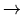HZ). Additional small contributions would come from ZZ or WW fusion processes (resulting in He+e- and H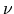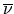final states respectively).

Using all of the available Electroweak measurements at the time of the Spring 1999 conferences, the Higgs mass was indirectly determined  to be mH = 71+75-42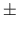5 GeV (central value and 68% C.L. errors) and mH < 220 GeV at 95% C.L. For data collected at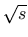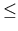183 GeV, the combined LEP direct search lower mass limit is mH > 89.7 GeV. The reconstructed mH in candidate events, from all final states, is a variable entering the calculation of confidence levels. Figures 1 and 2 show MC Higgs mass distributions ( mH = 70 GeV) for various final states, taken from an ALEPH analysis .

The Hl+l- (l = e or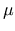) final state represents 6.7% of the Higgsstrahlung cross-section. It is characterised by two oppositely charged isolated leptons, where the mass of the lepton pair is close to the Z mass. The recoil mass can be calculated from the ll (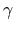) system, where therefers to any final state radiation that is emitted. Due to the high resolution of charged leptons (see Table 2), the reconstructed Higgs mass also has high resolution.

The missing energy channel Hrepresents 20% of the Higgsstrahlung cross-section. In the event of a signal, there would be large missing energy, a missing mass near the Z mass, acoplanar jets and the possibility of b-tagging these jets. The Higgs mass would have to be reconstructed from the di-jet mass, so it has larger width and a longer low-mass tail in the reconstructed mass distribution compared to that of the Hl+l- channel. This is clearly apparent in Figure 1. Forward calorimetry that is as hermetic as possible plays an important part in the event selection, contributing to the rejection of Z, Weand Zee events.

The four-jet channel, HZb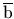q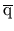, comprises 64.6% of the Higgsstrahlung cross-section. A signal in this final state would contain four isolated jets. A kinematic fit can be employed, after which the key trick is to plot m12 + m34 - mZ. The subscripts refer to jet pairings options. In Figure 2 the subsequent low mass tail due to jet combinatorics can be seen, which exists even though only the combination for which m12 is closest to the nominal Z mass is shown.Next: Summary Up: pre Previous: mW Results
latex2html conversion by www person on 1999-12-05